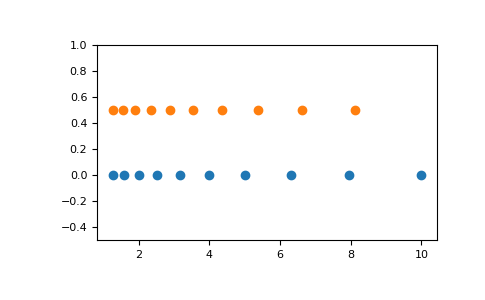# numpy.logspace¶

`numpy.``logspace`(start, stop, num=50, endpoint=True, base=10.0, dtype=None, axis=0)[source]

Return numbers spaced evenly on a log scale.

In linear space, the sequence starts at `base ** start` (base to the power of start) and ends with `base ** stop` (see endpoint below).

Changed in version 1.16.0: Non-scalar start and stop are now supported.

Parameters: start : array_like `base ** start` is the starting value of the sequence. stop : array_like `base ** stop` is the final value of the sequence, unless endpoint is False. In that case, `num + 1` values are spaced over the interval in log-space, of which all but the last (a sequence of length num) are returned. num : integer, optional Number of samples to generate. Default is 50. endpoint : boolean, optional If true, stop is the last sample. Otherwise, it is not included. Default is True. base : float, optional The base of the log space. The step size between the elements in `ln(samples) / ln(base)` (or `log_base(samples)`) is uniform. Default is 10.0. dtype : dtype The type of the output array. If `dtype` is not given, infer the data type from the other input arguments. axis : int, optional The axis in the result to store the samples. Relevant only if start or stop are array-like. By default (0), the samples will be along a new axis inserted at the beginning. Use -1 to get an axis at the end. New in version 1.16.0. samples : ndarray num samples, equally spaced on a log scale.

`arange`
Similar to linspace, with the step size specified instead of the number of samples. Note that, when used with a float endpoint, the endpoint may or may not be included.
`linspace`
Similar to logspace, but with the samples uniformly distributed in linear space, instead of log space.
`geomspace`
Similar to logspace, but with endpoints specified directly.

Notes

Logspace is equivalent to the code

```>>> y = np.linspace(start, stop, num=num, endpoint=endpoint)
...
>>> power(base, y).astype(dtype)
...
```

Examples

```>>> np.logspace(2.0, 3.0, num=4)
array([  100.        ,   215.443469  ,   464.15888336,  1000.        ])
>>> np.logspace(2.0, 3.0, num=4, endpoint=False)
array([ 100.        ,  177.827941  ,  316.22776602,  562.34132519])
>>> np.logspace(2.0, 3.0, num=4, base=2.0)
array([ 4.        ,  5.0396842 ,  6.34960421,  8.        ])
```

Graphical illustration:

```>>> import matplotlib.pyplot as plt
>>> N = 10
>>> x1 = np.logspace(0.1, 1, N, endpoint=True)
>>> x2 = np.logspace(0.1, 1, N, endpoint=False)
>>> y = np.zeros(N)
>>> plt.plot(x1, y, 'o')
[<matplotlib.lines.Line2D object at 0x...>]
>>> plt.plot(x2, y + 0.5, 'o')
[<matplotlib.lines.Line2D object at 0x...>]
>>> plt.ylim([-0.5, 1])
(-0.5, 1)
>>> plt.show()
```numpy.linspace

numpy.geomspace